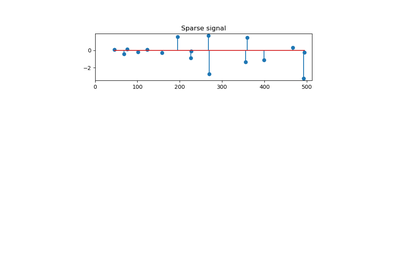# 3.2.4.1.8. `sklearn.linear_model`.OrthogonalMatchingPursuitCV¶

class `sklearn.linear_model.``OrthogonalMatchingPursuitCV`(copy=True, fit_intercept=True, normalize=True, max_iter=None, cv=None, n_jobs=1, verbose=False)[source]

Cross-validated Orthogonal Matching Pursuit model (OMP)

Read more in the User Guide.

Parameters: copy : bool, optional Whether the design matrix X must be copied by the algorithm. A false value is only helpful if X is already Fortran-ordered, otherwise a copy is made anyway. fit_intercept : boolean, optional whether to calculate the intercept for this model. If set to false, no intercept will be used in calculations (e.g. data is expected to be already centered). normalize : boolean, optional, default True This parameter is ignored when `fit_intercept` is set to False. If True, the regressors X will be normalized before regression by subtracting the mean and dividing by the l2-norm. If you wish to standardize, please use `sklearn.preprocessing.StandardScaler` before calling `fit` on an estimator with `normalize=False`. max_iter : integer, optional Maximum numbers of iterations to perform, therefore maximum features to include. 10% of `n_features` but at least 5 if available. cv : int, cross-validation generator or an iterable, optional Determines the cross-validation splitting strategy. Possible inputs for cv are: None, to use the default 3-fold cross-validation, integer, to specify the number of folds. An object to be used as a cross-validation generator. An iterable yielding train/test splits. For integer/None inputs, `KFold` is used. Refer User Guide for the various cross-validation strategies that can be used here. n_jobs : integer, optional Number of CPUs to use during the cross validation. If `-1`, use all the CPUs verbose : boolean or integer, optional Sets the verbosity amount intercept_ : float or array, shape (n_targets,) Independent term in decision function. coef_ : array, shape (n_features,) or (n_targets, n_features) Parameter vector (w in the problem formulation). n_nonzero_coefs_ : int Estimated number of non-zero coefficients giving the best mean squared error over the cross-validation folds. n_iter_ : int or array-like Number of active features across every target for the model refit with the best hyperparameters got by cross-validating across all folds.

Methods

 `fit`(X, y) Fit the model using X, y as training data. `get_params`([deep]) Get parameters for this estimator. `predict`(X) Predict using the linear model `score`(X, y[, sample_weight]) Returns the coefficient of determination R^2 of the prediction. `set_params`(**params) Set the parameters of this estimator.
`__init__`(copy=True, fit_intercept=True, normalize=True, max_iter=None, cv=None, n_jobs=1, verbose=False)[source]
`fit`(X, y)[source]

Fit the model using X, y as training data.

Parameters: X : array-like, shape [n_samples, n_features] Training data. y : array-like, shape [n_samples] Target values. Will be cast to X’s dtype if necessary self : object returns an instance of self.
`get_params`(deep=True)[source]

Get parameters for this estimator.

Parameters: deep : boolean, optional If True, will return the parameters for this estimator and contained subobjects that are estimators. params : mapping of string to any Parameter names mapped to their values.
`predict`(X)[source]

Predict using the linear model

Parameters: X : {array-like, sparse matrix}, shape = (n_samples, n_features) Samples. C : array, shape = (n_samples,) Returns predicted values.
`score`(X, y, sample_weight=None)[source]

Returns the coefficient of determination R^2 of the prediction.

The coefficient R^2 is defined as (1 - u/v), where u is the residual sum of squares ((y_true - y_pred) ** 2).sum() and v is the total sum of squares ((y_true - y_true.mean()) ** 2).sum(). The best possible score is 1.0 and it can be negative (because the model can be arbitrarily worse). A constant model that always predicts the expected value of y, disregarding the input features, would get a R^2 score of 0.0.

Parameters: X : array-like, shape = (n_samples, n_features) Test samples. y : array-like, shape = (n_samples) or (n_samples, n_outputs) True values for X. sample_weight : array-like, shape = [n_samples], optional Sample weights. score : float R^2 of self.predict(X) wrt. y.
`set_params`(**params)[source]

Set the parameters of this estimator.

The method works on simple estimators as well as on nested objects (such as pipelines). The latter have parameters of the form `<component>__<parameter>` so that it’s possible to update each component of a nested object.

Returns: self :

## 3.2.4.1.8.1. Examples using `sklearn.linear_model.OrthogonalMatchingPursuitCV`¶Orthogonal Matching Pursuit